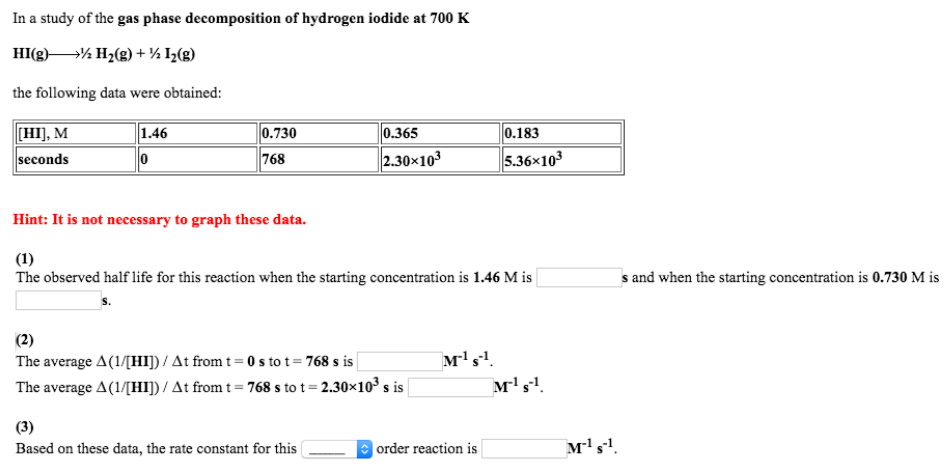# Problem: In a study of the gas phase decomposition of hydrogen iodide at 700 K.HI (g) → 1/2H2 (g) + 1/2I2 (g) the following data were obtained: (1) The observed half life for this reaction when the starting concentration is 1.46 M is ______ s and when the starting concentration is 0.730 M is _______ s. (2) The average Δ(1/[HI])/Δt from t = 0 s to t = 768 s is _______ M-1 s-1. The average Δ(1/[HI])/Δt from t = 768 s to t = 2.30 x 103 s is ________ M-1 s-1. (3) Based on these data, the rate constant for this order reaction is ________ M-1 s-1.

###### FREE Expert Solution
88% (303 ratings)###### Problem Details

In a study of the gas phase decomposition of hydrogen iodide at 700 K.

HI (g) → 1/2H2 (g) + 1/2I2 (g) the following data were obtained:

(1) The observed half life for this reaction when the starting concentration is 1.46 M is ______ s and when the starting concentration is 0.730 M is _______ s.

(2) The average Δ(1/[HI])/Δt from t = 0 s to t = 768 s is _______ M-1 s-1. The average Δ(1/[HI])/Δt from t = 768 s to t = 2.30 x 103 s is ________ M-1 s-1

(3) Based on these data, the rate constant for this order reaction is ________ M-1 s-1.